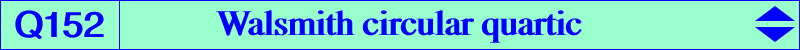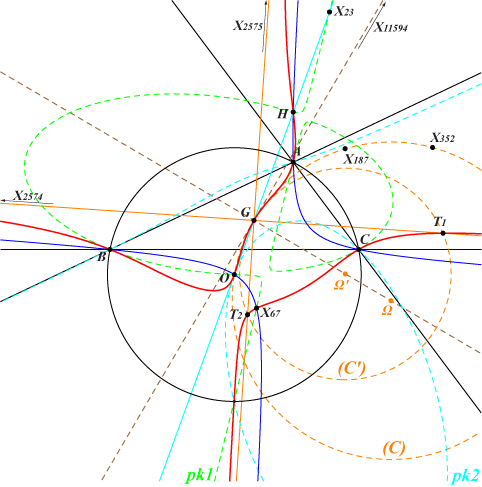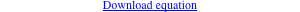too complicated to be written here. Click on the link to download a text file.X(2), X(3), X(4), X(67), X(2574), X(2575) anti-points, see Table 77 other points belowThe Walsmith triangle WT is defined in page K1091. It is orthologic to the circumcevian triangle ccv(P) of any P on the Walsmith circular quartic Q152. The third polar of X(2) splits into the line at infinity (L∞) and the circle (C) passing through X(2), X(3), X(187), X(352) with center Ω. Hence, for any M on these two curves, the polar line of M in Q152 passes through X(2). It follows that Q152 has two isotropic asymptotes passing through X(2) which is the singular focus of Q152 and two real asymptotes parallel at X(2) to those of the Jerabek hyperbola (J) or the Stammler hyperbola (S). The fourth points T1, T2 of Q152 on these real asymptotes are the end-points of the diameter of a circle (C') with center Ω', passing through X(2) and surosculating Q152 at this point. Note that Ω' is homothetic to Ω under h(X2, 2/3). The tangent to Q152 at X(2) is parallel to the line {23, 183} and X(2), Ω, Ω' are collinear on the perpendicular at X(2) to this same line i.e. the normal at X(2) to Q152. Q152 meets the circumcircle (O) at A, B, C, the circular points at infinity and three remaining points which lie : • on the rectangular hyperbola (H) with center X(1495) passing through X(3), X(23), X(110), X(1177), X(2574), X(2575), X(2930), etc. The two points of (H) on the line passing through X(2), X(67) lie on Q152. • on the cubics pk1= pK(X6, X23), pk2 = pK(X187, X3), pk3 = pK(X3 x X1177, X1177). These three pKs belong to a same pencil and there is no other pK in this pencil. Q152 may be (loosely) written (L∞) U (pk1) – 3 (J) U (O) and (L∞) U (pk2) – 3 (S) U (O). The tangents at A, B, C to Q152 concur at X(67). The tangent at A meets the perpendicular bisector of BC at A1 on Q152. B1 and C1 being defined similarly, the triangle A1B1C1 is homothetic at X(5094) to the orthic triangle. The tangent at X(3) is the Euler line. *** Some points and coordinates • Ω = a^2 (b^2-c^2) (4 a^6-10 a^4 b^2+8 a^2 b^4-2 b^6-10 a^4 c^2+15 a^2 b^2 c^2-b^4 c^2+8 a^2 c^4-b^2 c^4-2 c^6) : : , SEARCH = -0.278073048284580, on the lines {3, 17414}, {182, 9044}, {187, 30491}, {351, 1499}, {512, 5926}, {523, 549}, {647, 2780}, {3288, 21733}, {8552, 8675}, {8704, 11621}, {11615, 30230}, {16235, 23878}. Ω = midpoint of {3, 17414} and {3288, 21733} = reflection of X(5926) in X(9126). • Ω' = (b^2-c^2) (5 a^8-13 a^6 b^2+11 a^4 b^4-3 a^2 b^6-13 a^6 c^2+18 a^4 b^2 c^2-2 a^2 b^4 c^2+b^6 c^2+11 a^4 c^4-2 a^2 b^2 c^4-2 b^4 c^4-3 a^2 c^6+b^2 c^6) : : , SEARCH = 0.691074231973186, on the lines {523, 5054}, {549, 17414}, {5215, 8704}, {5996, 9126}. Ω' = 2 X + X = X + 2 X. Ω, Ω' are now X(32231), X(32232) in ETC (2019-04-30). *** Orthologic centers of WT and ccv(P) • P = X(2) : X(1316) and X(110). • P = X(3) : X(125) and intersection of the lines {3,67}, {20,2781}, {110,858}, SEARCH = 7.577090880093897. • P = X(4) : X(110) and intersection of the lines {4,542}, {24,2930}, {54,67}, {110,468}, SEARCH =2.667709987824752. • P = X(67) : a point on the line {2,98}, SEARCH = 2.946520901345807 and X(67) itself. These three points are now X(32233), X(32234), X(32235) in ETC (2019-04-30).